# Newtons Laws of Motion Newtons First Law An

• Slides: 17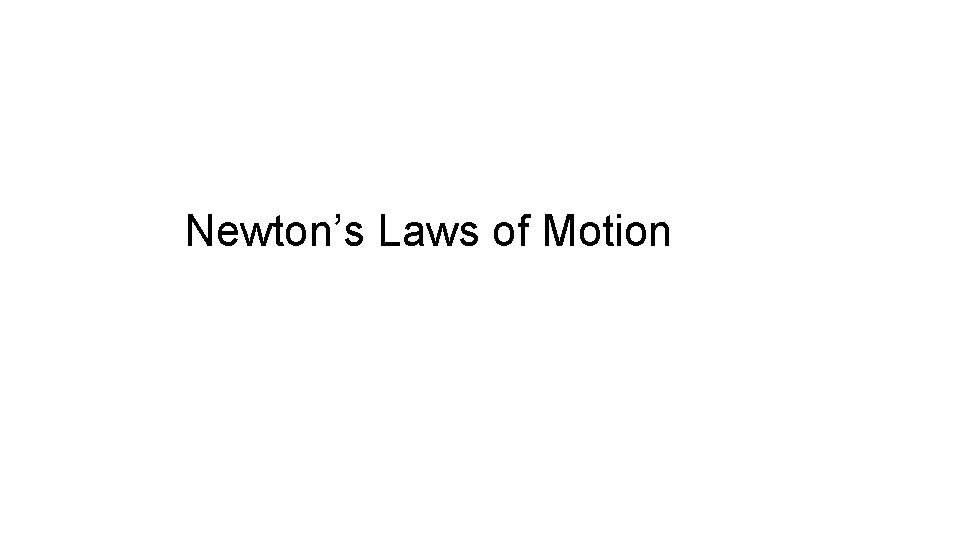Newton’s Laws of Motion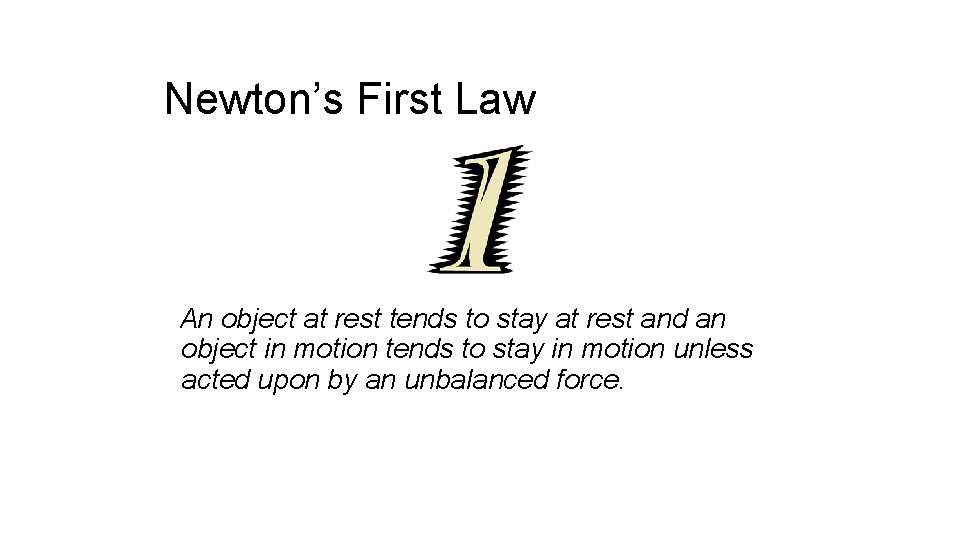Newton’s First Law An object at rest tends to stay at rest and an object in motion tends to stay in motion unless acted upon by an unbalanced force.What does this mean? Basically, an object will “keep doing what it was doing” unless acted on by an unbalanced force. If the object was sitting still, it will remain stationary. If it was moving at a constant velocity, it will keep moving. It takes force to change the motion of an object.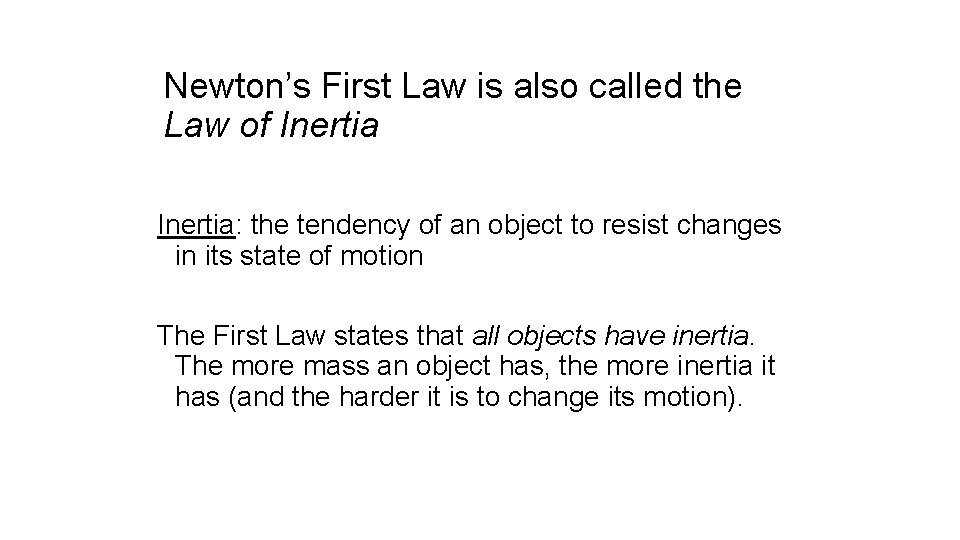Newton’s First Law is also called the Law of Inertia: the tendency of an object to resist changes in its state of motion The First Law states that all objects have inertia. The more mass an object has, the more inertia it has (and the harder it is to change its motion).What is meant by unbalanced force? If the forces on an object are equal and opposite, they are said to be balanced, and the object experiences no change in motion. If they are not equal and opposite, then the forces are unbalanced and the motion of the object changes.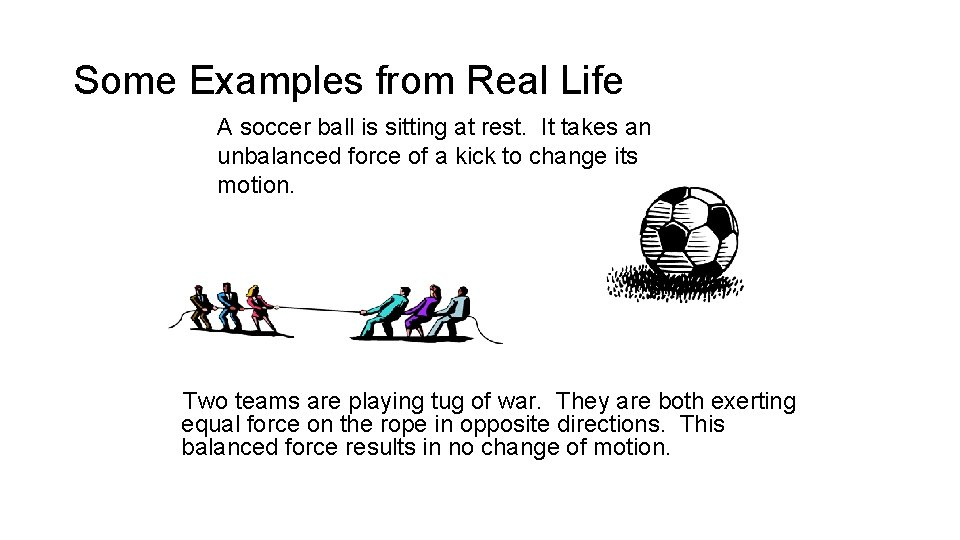Some Examples from Real Life A soccer ball is sitting at rest. It takes an unbalanced force of a kick to change its motion. Two teams are playing tug of war. They are both exerting equal force on the rope in opposite directions. This balanced force results in no change of motion.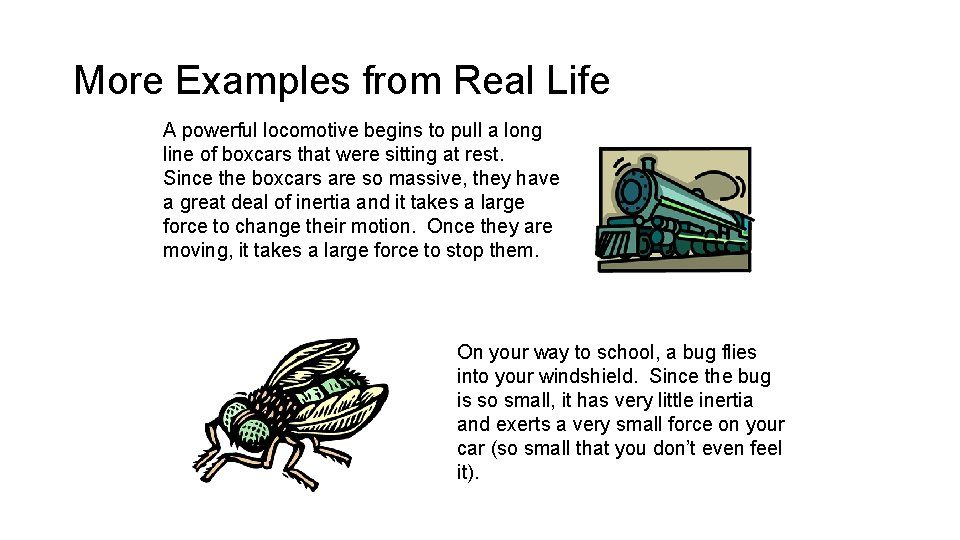More Examples from Real Life A powerful locomotive begins to pull a long line of boxcars that were sitting at rest. Since the boxcars are so massive, they have a great deal of inertia and it takes a large force to change their motion. Once they are moving, it takes a large force to stop them. On your way to school, a bug flies into your windshield. Since the bug is so small, it has very little inertia and exerts a very small force on your car (so small that you don’t even feel it).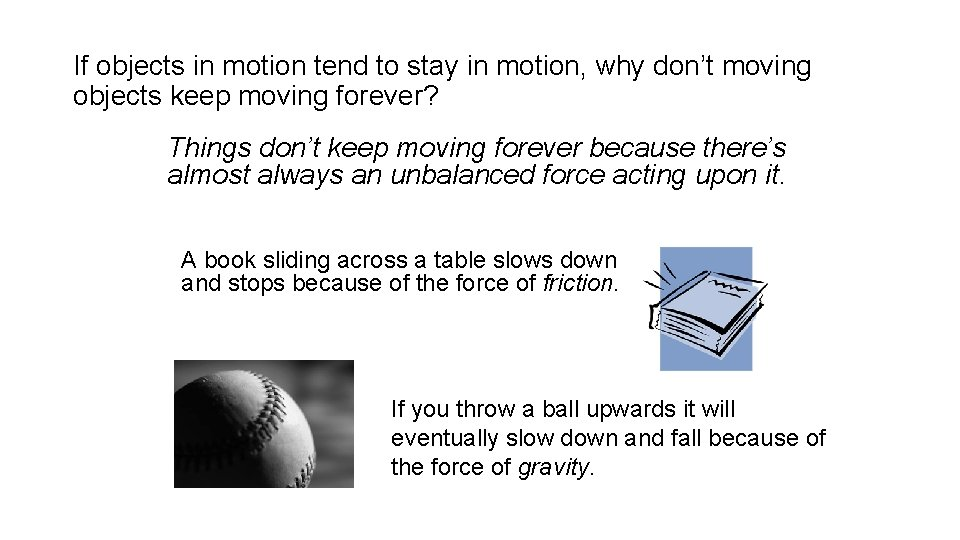If objects in motion tend to stay in motion, why don’t moving objects keep moving forever? Things don’t keep moving forever because there’s almost always an unbalanced force acting upon it. A book sliding across a table slows down and stops because of the force of friction. If you throw a ball upwards it will eventually slow down and fall because of the force of gravity.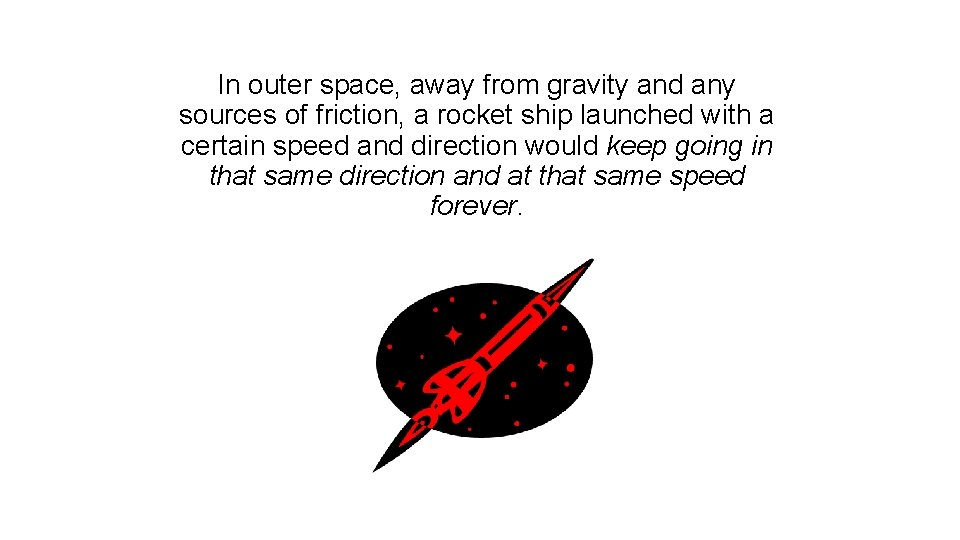In outer space, away from gravity and any sources of friction, a rocket ship launched with a certain speed and direction would keep going in that same direction and at that same speed forever.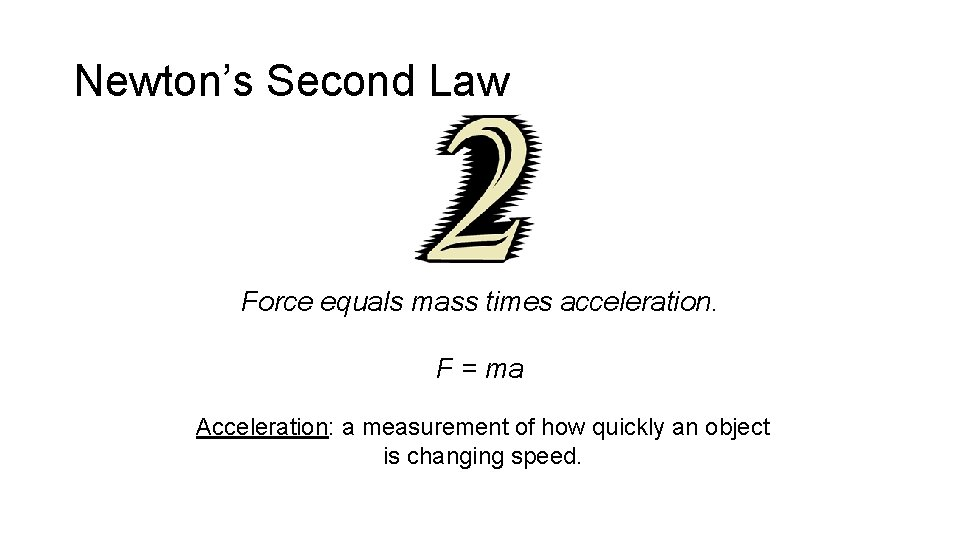Newton’s Second Law Force equals mass times acceleration. F = ma Acceleration: a measurement of how quickly an object is changing speed.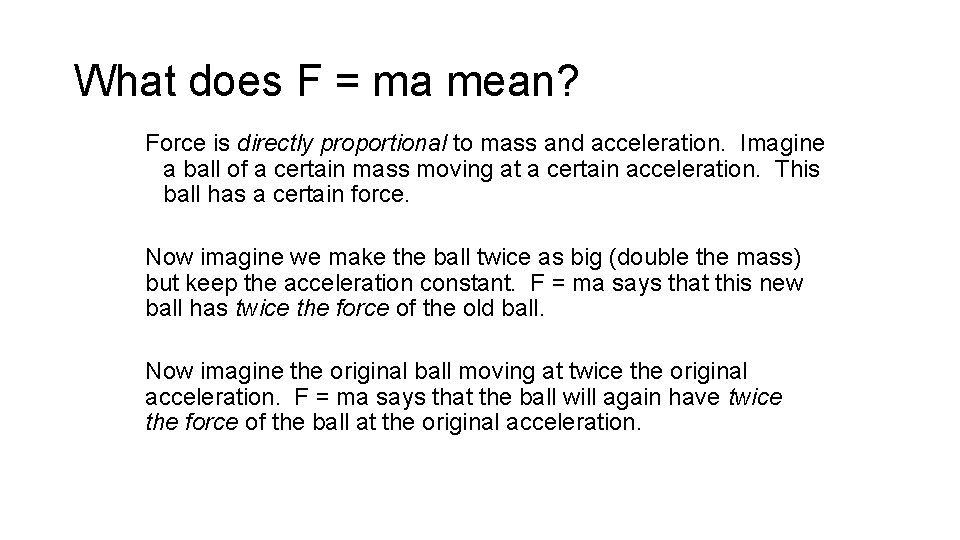What does F = ma mean? Force is directly proportional to mass and acceleration. Imagine a ball of a certain mass moving at a certain acceleration. This ball has a certain force. Now imagine we make the ball twice as big (double the mass) but keep the acceleration constant. F = ma says that this new ball has twice the force of the old ball. Now imagine the original ball moving at twice the original acceleration. F = ma says that the ball will again have twice the force of the ball at the original acceleration.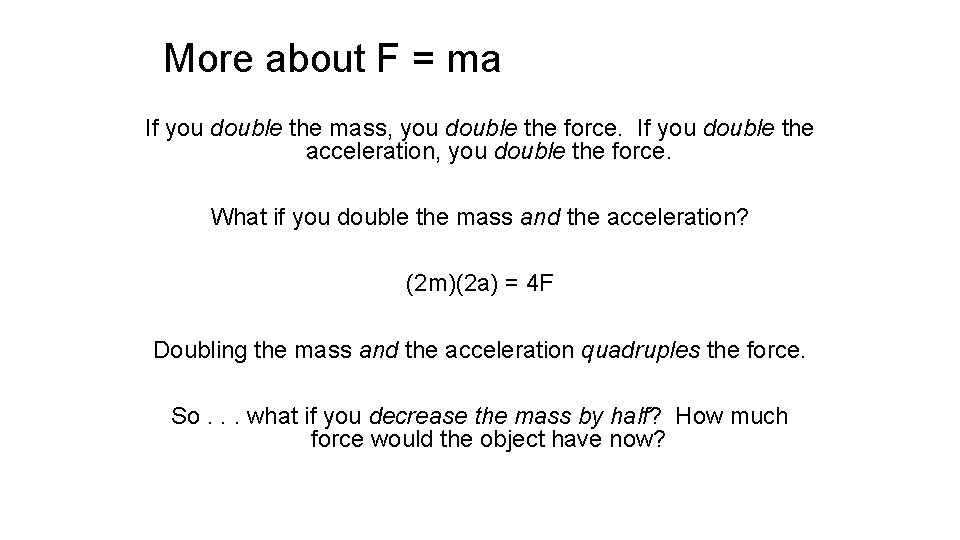More about F = ma If you double the mass, you double the force. If you double the acceleration, you double the force. What if you double the mass and the acceleration? (2 m)(2 a) = 4 F Doubling the mass and the acceleration quadruples the force. So. . . what if you decrease the mass by half? How much force would the object have now?What does F = ma say? F = ma basically means that the force of an object comes from its mass and its acceleration. Something very massive (high mass) that’s changing speed very slowly (low acceleration), like a glacier, can still have great force. Something very small (low mass) that’s changing speed very quickly (high acceleration), like a bullet, can still have a great force. Something very small changing speed very slowly will have a very weak force.Newton’s Third Law For every action there is an equal and opposite reaction.What does this mean? For every force acting on an object, there is an equal force acting in the opposite direction. Right now, gravity is pulling you down in your seat, but Newton’s Third Law says your seat is pushing up against you with equal force. This is why you are not moving. There is a balanced force acting on you– gravity pulling down, your seat pushing up.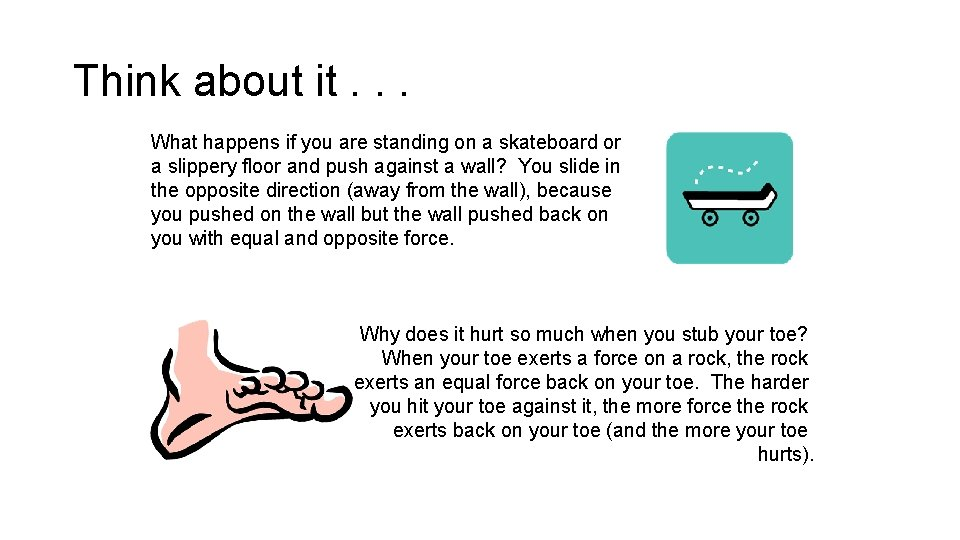Think about it. . . What happens if you are standing on a skateboard or a slippery floor and push against a wall? You slide in the opposite direction (away from the wall), because you pushed on the wall but the wall pushed back on you with equal and opposite force. Why does it hurt so much when you stub your toe? When your toe exerts a force on a rock, the rock exerts an equal force back on your toe. The harder you hit your toe against it, the more force the rock exerts back on your toe (and the more your toe hurts).Review Newton’s First Law: Objects in motion tend to stay in motion and objects at rest tend to stay at rest unless acted upon by an unbalanced force. Newton’s Second Law: Force equals mass times acceleration (F = ma). Newton’s Third Law: For every action there is an equal and opposite reaction.